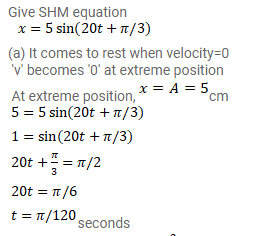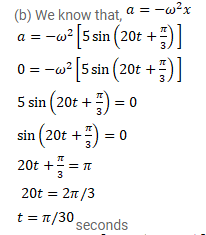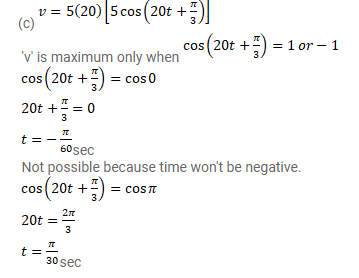# The equation of motion of a particle started at

Question:

The equation of motion of a particle started at $\mathrm{t}=0$ is given by $x=5 \sin (20 t+\pi / 3)$ where $\mathrm{x}$ is in centimeter and t in second. What does the particle

(a) first come to rest.

(b) First have zero acceleration.

(c) First have maximum speed?

Solution: